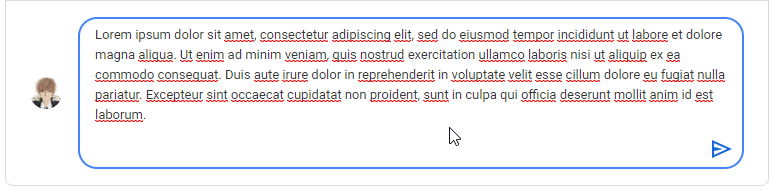# How would I make a textbox increase a size when one column is full with chars and then goes to the next line?

I was trying to use Basic admin essentials way of resizing the response box in the PM UI. Suddenly, it goes wrong when using the offsets and scales.

Using google classroom comment textbox as an example.you can use :GetTextSize, pretty much all you’re doing is setting the size with the returned Vector2.Y value, if you do it correctly you get something like this:

1 Like

I’ve tried doing this, but then it would do the same thing.

``````local textbox = script.Parent.TextBox
local frame = script.Parent

textbox.Changed:Connect(function(prot)
if prot == 'TextBounds' then
local textsize = game:GetService'TextService':GetTextSize(textbox.Text, textbox.TextSize, textbox.Font, Vector2.new(300, 47))
frame.Size = UDim2.new(0, 300, 0, textsize.Y+22)
frame.Position = UDim2.new(0.461, 0, 0.446, -frame.Size.Y.Offset)
end
end)
``````

try using `textbox:GetPropertyChangedSignal('Text')`

1 Like

Not really helpful. Have you found other ways of knowing how to make your textbox work like that?

something like this

``````local textbox = script.Parent.TextBox
local frame = script.Parent
local ts = game:GetService("TextService")

textbox:GetPropertyChangedSignal("Text"):Connect(function()
local a = ts:GetTextSize(textbox.Text,textbox.TextSize,textbox.Font,Vector2.new(250,50)) -- you can fool around with this
textbox.Size = UDim2.new(0.2,a.X,0,a.Y) -- and this
end)
``````
4 Likes